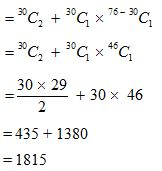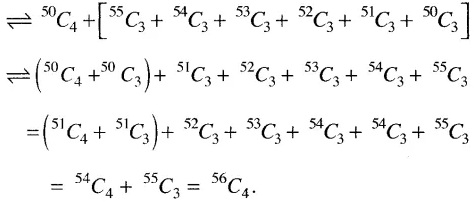# JEE Main Permutations and Combinations Previous Year Questions With Solutions

JEE Main Mathematics Permutations and Combinations previous year papers questions with solutions are available here. Find the permutation and combination questions from the previous years of IIT JEE Main in this article along with a detailed solution for each question. These questions include all the important concepts and formulae.

## JEE Main Maths Permutations and Combinations Previous Year Questions With Solutions

Question 1: A letter lock consists of three rings, each marked with ten different letters. In how many ways is it possible to make an unsuccessful attempt to open the lock?

Solution:

Two rings may have the same letter at a time, but the same ring cannot have two letters at a time. Therefore, we must proceed ring wise. Each of the three rings can have any one of the 10 different letters in 10 ways.

Therefore, the total number of attempts = 10 × 10 × 10 = 1000.

But out of these 1000 attempts, only one attempt is successful.

Therefore, the required number of unsuccessful attempts = 1000 – 1 = 999.

Question 2: The total number of positive integral solution for x, y, z such that x* y * z = 24, is ________.

Solution:

We have,

x* y * z = 24

x* y * z = 23 × 31

The number of ways of distributing ‘n’ identical balls into ‘r’ different boxes is (n + r − 1)C(r − 1)

Here we have to group 4 numbers into three groups

Number of integral positive solutions

= (3 + 3 − 1)C(3 − 1) × (1+ 3 − 1)C(3 − 1)

= 5C2 × 3C2

= 30

Question 3: Find the total number of signals that can be made by five flags of a different color when any number of them may be used in any signal.

Solution:

Case I: When only one flag is used. No. of signals made = 5P1 = 5.

Case II: When only two flags are used. Number of signals made = 5P2 = 5 * 4 = 20.

Case III: When only three flags are used. Number of signals is made = 5P3 = 5 * 4 * 3 = 60.

Case IV : When only four flags are used. Number of signals made = 5P4 = 5 * 4 * 3 * 2 = 120.

Case V : When five flags are used. Number of signals made = 5P5 = 5! = 120.

Hence, required number = 5 + 20 + 60 + 120 + 120 = 325.

Question 4: Prove that if each of the ‘m’ points in one straight line is joined to each of the n points on the other straight line, excluding the points on the given two lines. The number of points of intersection of these lines is $\frac{1}{4}$ mn (m-1 ) (n-1).

Solution:

To get one point of intersection we need two points on the first line and two points on the second line. These can be selected out of n-points in nC2 ways and for m points in mC2 ways.

Therefore, the required number = mC2 × nC2 = (m(m-1))/2!  x  (n(n-1))/2! = $\frac{1}{4}$ m n (m – 1)(n – 1)

Question 5: There are ten points in a plane. Of these ten points, four points are in a straight line, and except for these four points, no other three points are in the same straight line. Find

(i) The number of straight lines formed.

(ii) The number of triangles formed.

(iii) The number of quadrilaterals formed by joining these ten points.

Solution:

(i) For a straight line, we need 2 points.

 No. of points selected out of 4 collinear points No. of points selected out of the remaining 6 points No. of straight-lines formed 0 2 4C0 × 6C2 = 15 1 1 4C1 × 6C1 = 24 2 0 1

(In the last case only one straight line is formed)

Therefore, the required number = 15 + 24 + 1 = 40

(ii) For a triangle, we need 3 points.

 No. of points selected out of 4 collinear points No. of points selected out of the remaining 6 points No. of triangles formed 0 3 4C0 × 6C3 = 20 1 2 4C1 × 6C2 = 60 2 1 4C2 × 6C1 = 36 3 0 0

(In last case number of triangles formed is 0)

Therefore, the required number = 20 + 60 + 36 + 0 = 116

(iii) For a quadrilateral, we need 4 points.

 No. of points selected out of 4 collinear points No. of points selected out of the remaining 6 points No. of quadrilaterals formed 0 4 4C0 × 6C4 = 15 1 3 4C1 × 6C3 = 80 2 2 4C2 × 6C2 = 90 3 1 0 4 0 0

Therefore, the required number = 15 + 80 + 90 = 185.

Question 6: Two numbers are chosen from 1, 3, 5, 7,… 147, 149 and 151 and multiplied together in all possible ways. The number of ways which will give the product a multiple of 5 is ______.

Solution:

In the given numbers 1, 3, 5, 7,…, 147, 148, 151 the numbers which are multiples of 5 are 5, 15, 25, 35, …, 145, which are an arithmetic sequence.

Tn = a + (n − 1) * d

145 = 5 + (n – 1) 10

n = 15

and if total number of terms in the given sequence is m, then

151 = 1 + (m – 1) ´ 2

m = 76

So, the number of ways in which product is a multiple of 5 = (both two numbers from 5, 10, 15, 20, 25, 30, 35, … , 150) or (one number from 5, 10, 15, 20, 25, 30, 35, … , 150 and one from remaining numbers)Question 7: If the letters of the word SACHIN are arranged in all possible ways, and these words are written in a dictionary, at what serial number does the word SACHIN appear?

Solution:

(1) Alphabetical order is A, C, H, I, N, S

No. of words starting with A – 5!

No. of words starting with C – 5!

No. of words starting with H – 5!

No. of words starting with I – 5!

No. of words starting with N – 5!

SACHIN-1

601.

Question 8: The value of 50C4 + $\sum_{r=1}^{6}$ 56-rC3 is ________.

Solution:Question 9: Assuming the balls to be identical except for the difference in colours, the number of ways in which one or more balls can be selected from 10 white, 9 green and 7 black balls is ______.

Solution:

p = 10, q = 9, r = 7

Total ways of selection = (p + 1) * (q + 1) * (r + 1) – 1

= [11 * 10 * 8] – 1

= 879

Question 10: From 6 different novels and 3 different dictionaries, 4 novels and a dictionary are to be selected and arranged in a row on the shelf such that the dictionary is in the middle. What is the number of such arrangements?

Solution:

4 novels can be selected from 6 novels in 6C4 ways. 1 dictionary can be selected from 3 dictionaries in 3C1 ways. As the dictionary selected is fixed in the middle, the remaining 4 novels can be arranged in 4! ways.

Hence, the required number of ways of arrangement = 6C4 * 3C1.* 4! = 1080

Question 11: Number of divisors of n = 38808 (except 1 and n) is _____.

Solution:

Since, 38808 = 8 × 4851

= 8 × 9 × 539

= 8 × 9 × 7 × 7 × 11

= 23 × 32 × 72 × 11

So, the number of divisors = (3 + 1) (2 + 1) (2 + 1) (1 + 1) = 72.

This includes two divisors 1 and 38808.

Hence, the required number of divisors = 72 – 2 = 70.

Question 12: An n-digit number is a positive number with exactly n digits. Nine hundred distinct n-digit numbers are to be formed using only the three digits 2, 5 and 7. The smallest value of n for which this is possible is _______.

Solution:

Since at any place, any of the digits 2, 5 and 7 can be used, the total number of such positive n-digit numbers are 3n. Since we have to form 900 distinct numbers, hence 3n ≥ 900 ⇒ n = 7.

Question 13: In how many ways can 15 members of a council sit around a circular table, when the Secretary is to sit on one side of the Chairman and the Deputy Secretary on the other side?

Solution:

Since the total members are 15, but one is to the left, because of circular condition. Therefore, the remaining members are 14, but three special members constitute a member. Therefore the required number of arrangements are 12 ! ×2, because the chairman remains between the two specified persons and the person can sit in two ways.

Question 14: In how many ways can 5 boys and 5 girls sit in a circle so that no two boys sit together?

Solution:

Since the total number of ways in which boys can occupy any place is (5 − 1)! = 4 ! and the 5 girls can sit accordingly in 5 ! ways. Hence the required number of ways is 4 ! ×5 !.

Question 15: A five-digit number divisible by 3 has to be formed using the numerals 0, 1, 2, 3, 4 and 5 without repetition. The total number of ways in which this can be done is ________.

Solution:

We know that a five-digit number is divisible by 3, if and only if the sum of its digits (= 15) is divisible by 3, therefore we should not use 0 or 3 while forming the five-digit numbers.

Now,

(i) In this case, we do not use 0; the five-digit number can be formed (from the digit 1, 2, 3, 4, 5) in 5P5 ways.

(ii) In this case, we do not use 3; the five-digit number can be formed (from the digit 0, 1, 2, 4, 5) in 6 ! −5! × 2 = 480 ways.

The total number of such 5 digit number = 5P5 + (5P5 4P4)

= 120 + 96

= 216.

## How Do IIT JEE Permutations and Combinations Question Papers Help Students?

IIT JEE aspirants can find Permutations and Combinations JEE Main Maths previous year questions with solutions here. This set of questions helps aspirants to understand the type of questions asked in the JEE exams. Download JEE Main previous year Permutations and Combinations questions PDFs for free and crack JEE exam.